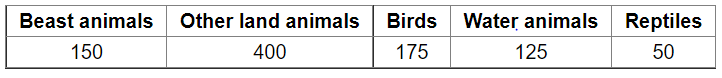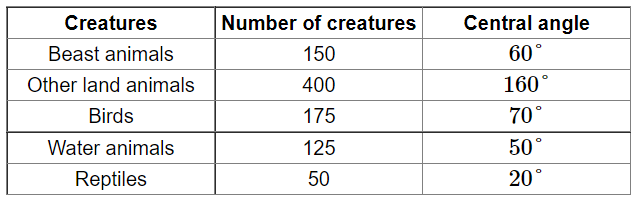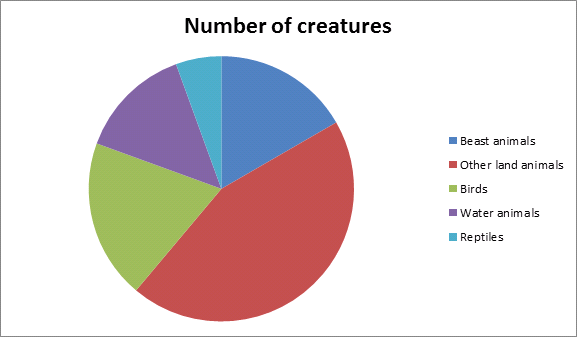# There are 900 creatures in a zoo as per list given below:

Question:

There are 900 creatures in a zoo as per list given below:Represent the above data by a pie chart.

Solution:

Total number of creatures $=900$

Central angle of each component $=\left(\frac{\text { number of creatures in each type }}{\text { total number of creatures }} \times 360\right)^{\circ}$

Calculation of central anglesConstruction of pie chart

Steps of construction:

1. Draw a circle of any convenient radius.

2. Draw a horizontal radius of this circle.

3. Draw sectors whose central angles are $60^{\circ}, 160^{\circ}, 70^{\circ}, 50^{\circ}$ and $20^{\circ}$.

4. Shade the sectors so obtained differently and label each one of them.

Thus, we obtain the required pie chart as shown in the figure below.